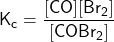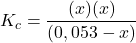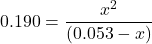## A 0.50 mol sample of COBr2 is transferred to a 9.50-L flask and heated until equilibrium is attained. Calculate the equilibrium concentratio

Question

A 0.50 mol sample of COBr2 is transferred to a 9.50-L flask and heated until equilibrium is attained. Calculate the equilibrium concentrations of each species.

in progress 0
6 months 2021-07-12T10:40:05+00:00 1 Answers 18 views 0

## Answers ( )

Equlibrium concentration for each species ae as follows:

[CO] = 0.043 mol/L

[Br₂] = 0.043 mol/L

[COBr₂] = 0.01 mol/L

Explanation:

Let take a look at the chemical equation taking place at equilibrium

COBr2(g) ⇄ CO(g) + Br2(g)

The concentration of COBr2 i.e.

[COBr2] = no of moles/volume

= 0.50 mol/9.50 L

[COBr2] = 0.0530 mol/L

At standard conditions

Kc for COBr2 = 0.190

Now, the ICE table for the above reaction can be computed as follows:

COBr2(g)              ⇄       CO(g)         +           Br2(g)

Initial               0.053                                   0                           0

Change             -x                                       +x                          +x

Equilibrium     (0.053 – x)                              x                           xx² = 0.190(0.053 – x)

x² = 0.01007 – 0.190x

x² + 0.190x – 0.01007 = 0